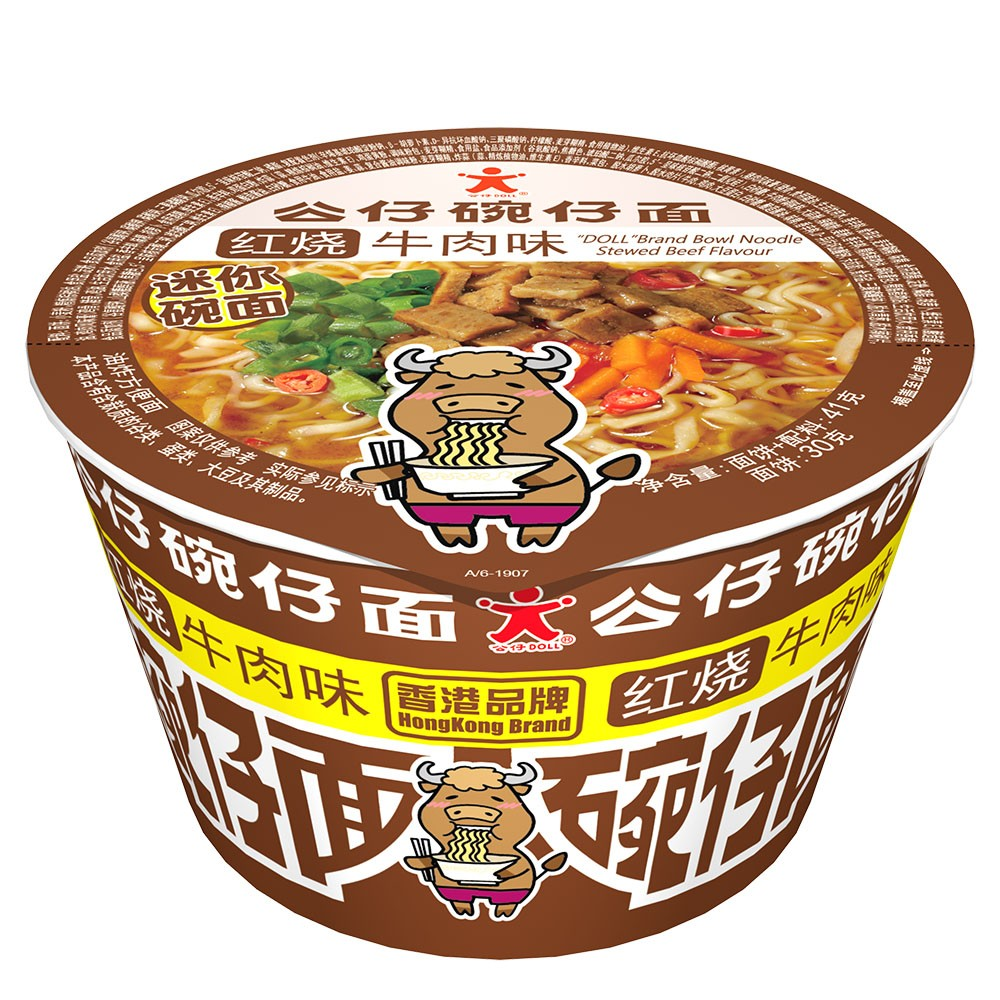• 请选择
• 请选择
• 请选择
• 请选择•# 【非自营】永南食品香港品牌公仔迷你碗仔面整箱18碗网红小杯面

• 售价：
￥42.00
• 建议零售价：
￥ 0.00
• 促销信息：
• 商品评分：
• 好评率：
100%
• 累计销量：
140件
• 配送至：
北京市
包邮
由格力董明珠店 发货
• 商品规格：
海鲜味1*1..... 风味排骨味1..... 滑蛋鸡肉味1..... 牛肉味1*1..... 鲜鱿海鲜味1..... 麻油味1*1.....
• 数量：

• 挂机
• 柜机
• 遥控器
• 空调过滤网
• 热风机
• 移动空调
• 风管机
• 天井机
• 线管器
• 单元柜机

• 电饭煲
• 电压力锅
• 除湿机
• 电风扇
• 电暖器
• 干衣机
• 加湿机
• 空气净化器
• 冷风扇
• 净水机
• 吸尘器
• 空气净化器滤芯
• 电火锅
• 净水机测试仪
• 燃气灶
• 油烟机
• 原汁机
• 垃圾桶
• 电水壶
• 电磁炉
• 蒸汽洗车机
• 消毒柜
• 净水机滤芯
• 净水机配件
• 储物罐
• 洗碗机
• 果汁杯
• 空气净化器滤网
• 蒸烤双能机
• 电饭盒
• 油烟机装饰板
• 电热水杯
• 火锅配件
• 空气炸锅
• 气泡水机
• 养生壶

• 对开门冰箱
• 多门冰箱
• 滚筒洗衣机
• 酒柜
• 三门冰箱
• 冰柜
• 双门冰箱
• 格力冰箱
• 单门冰箱
• 波轮洗衣机
• 冰箱除味器

• 善水方
• 水之韵
• 智能回水装置
• 舒智
• 御尙
• 海洋之梦
• 水之逸
• 润之恋
• 全能王-舒铂
• 厨享
• 水之沁
• 燃气热水器
• 沐尊
• 舒之恋

• 格力色界手机
• 格力二代
• 手机充电宝
• 格力三代
• 5G手机

• 厨房空调
• GMV-舒睿
• GMV-智睿
• GMV Tops
• 天井机
• 寐享风管机
• 淳享
• 舒享家
• GMV雅居
• 风管机
• 离心机系列
• 单元机系列
• 多联机系列
• 精密空调系列
• 特种机系列
• 新风净化系列
• 螺杆机系列
• 模块机系列
• 冷冻冷藏
• 清洁热水系列
• 终端空气处理系列
• 多联机内机

• 智能门锁
• 智能潮品
• 玲锐系列
• 空调伴侣

• 口罩
• 护眼镜
• 移动检测车
• 消毒液制造机
• 水牙线

• 漆包铜圆/扁线
• 铜包铝、铝漆包线
• 电线
• 电容器
• 系统平台
• 硬件产品
• 工业终端设备
• 螺杆压缩机
• 涡旋压缩机
• 活塞压缩机
• 转子压缩机
• 家电用电机
• 工业用电机
• 机器人乐队

• 设备

• 消费电子类

• 酒水
• 茶叶
• 面食
• 休闲食品
• 饮料
• 浓缩饮料
• 乳制饮品
• 白酒
• 猪肉脯
• 饼干
• 蛋卷
• 灰枣
• 蔬菜蛋品

• 潮流精品
• 珠宝
• 酒店

• 汽车

• 客厅专享
• 厨房专享
• 主卧·厅专享
• 两室一厅
• 两室两厅
• 三室两厅
• 四室两厅
• 全屋新风

• 精选大米
• 食用油
• 面粉
• 面条
• 公仔面
• 组合套装
• 方便面
• 碱水面
• 热干面
• 南北干货
• 方便速食
• 调味

• 水龙头
• 洗碗池
• 花洒
• 坐便器
• 浴室柜

• 空调清洗

• 钛酸锂电池

• 通信基站储能
• 集装箱式储能

• 罗西尼

• 滋补品
• 保健品

• 文创礼品

• 国内旅游
• 酒店

• 内衣

• 乐器
• 奶嘴奶瓶
• 水杯/水壶

• 男装/女装

• 面膜
• 口腔护理

*我的邮箱：
*手机：
*采购数量：